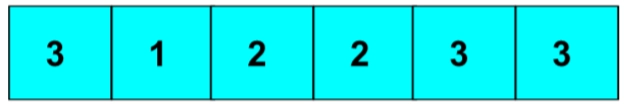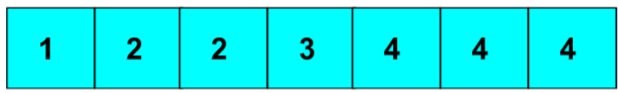New update is available. Click here to update.
Last Updated: Mar 6, 2023
Medium

# Minimum Number of Distinct Elements after Removing M itemsAuthor Abhishek Nayak0 upvotes

## Introduction

In this blog, we will discuss a problem in which we have to print the Minimum number of distinct elements after removing m items. We will be using the Hashmap data structure for solving the question.

A HashMap is a data structure that can map certain keys to specific values. The keys and values could be anything. Each data value has a unique index value in a hash table, which stores data in an array format. If we know the index of the needed data, data access becomes very fast. As a result, it develops into a data structure in which insertion and search operations, regardless of the size of the data, are very fast.

## Problem Statement

Ninja has given you an array of items, a number m. The i-th index element shows the item's id. Your task is removing m elements until just minimum distinct elements are left. Print the total count of the minimum number of distinct elements left after removing M items.

### Sample Examples

Example 1

InputEnter the value of m : 3

Output

``1``

Explanation: We have to remove 3 elements from the array so we start removing the elements with the least occurrence, so we remove 1 and both 2. Hence we are left with minimum distinct element 3 i.e., 1.

Example 2

InputEnter the value of m : 2

Output

``2``

Explanation: We have to remove 2 elements from the array so we start removing the elements with the least occurrence, so we remove 1 and 3. Hence we are left with minimum distinct elements 2 and 4 i.e., 2.

## Approach

The idea is to store each element's occurrence in a hash and then count the occurrence of each frequency in a hash again. Then we will sort the frequency of occurrences of each element in increasing order and will start removing the element whose frequency is less or equal to m.

### Algorithm

1. Create a hashmap to store the occurrence of elements.
2. Count the occurrence of all elements and store them in the hash.
3. Sort the hash in increasing order.
4. Remove the elements whose frequency is less than m from the hashmap.
5. Print the number of elements present in the hash.

### Implementation in C++

``````#include <bits/stdc++.h>
#include <algorithm>
using namespace std;

#define pb push_back

int hash_fun(int arr[], int n, int m)
{
int temp = 0;
// hashmap for storing the occurence
unordered_map<int, int> mp;
vector<pair<int, int>> v;

for (int i = 0; i < n; i++)
mp[arr[i]]++;

// use vector to store the pair key with its occurrence
for (auto i = mp.begin(); i != mp.end(); i++)
v.pb(make_pair(i->second, i->first));

sort(v.begin(), v.end()); // sorting the vector

int vec_size = v.size();

for (int i = 0; i < vec_size; i++)
{
// Remove the value if it is less than or equal to m
// else return the remaining size
if (v[i].first <= m)
{
m = m - v[i].first;
temp++;
}
else
return vec_size - temp;
}
return vec_size - temp;
}

int main()
{
int m;
int arr[] = {3, 1, 2, 2, 3, 3};
int size = sizeof(arr) / sizeof(arr);

cout << "Enter the value of m : ";
cin >> m;
cout << endl;

cout << hash_fun(arr, size, m);
return 0;
}``````

Input

``Enter the value of m : 3``

Output

``1``

#### Time Complexity

The time complexity of the above approach is O(NlogN) because we have used sorting, which marks the upper bound for time complexity.

#### Space Complexity

The space complexity of the approach is O(N), as we are storing the elements as a key in the hashmap.

### What is HashMap data structure?

A HashMap is a data structure that can map certain keys to a specific value. The values and keys could be anything.

### Is sort in C++ STL?

A C++ Standard Template Library(STL) includes a built-in function called sort(). The elements in the range can be sorted using this function in either ascending or descending order.

### What is the time complexity of sort function in C++ STL?

The worst-case time complexity of sort() is in O(n log n), where n is the number of sorted elements.

## Conclusion

In this article, we have extensively discussed the problem of printing the minimum number of distinct elements after removing m items. We solved the problem using the hashmap data structure and discussed its time as well as space complexity.

We hope that this blog has helped you enhance your knowledge about the count of minimum distinct elements and if you like to learn more, check out our articles Implementation of HashMapSorting Based ProblemsSTL in C++, and many more on our Website.

Recommended Problems -

Refer to our Guided Path on CodeStudio to upskill yourself in Data Structures and AlgorithmsCompetitive ProgrammingJavaScriptSystem Design, and many more! If you want to test your competency in coding, you may check out the mock test series and participate in the contests hosted on CodeStudio! But if you have just started your learning process and are looking for questions asked by tech giants like Amazon, Microsoft, Uber, etc; you must look at the problemsinterview experiences, and interview bundle for placement preparations.

Do upvote our blogs if you find them helpful and engaging!

Happy Learning!

Codekaze-June23 India's Biggest Tech Hiring Challenge is LIVE!Go on top